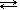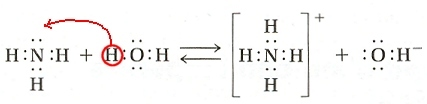# Chemistry 30

Redox Reactions: Module Index | Practice Problems | Assignments | Student Lab | Research Ideas | Teacher Resources## Acids & Bases

### 1.4 Brønsted-Lowry Theory of Acids & Bases

That lack of an OH- group in ammonia is a bit of a problem at first glance, but let's take a closer look at what happens when NH3 is added to water:

 NH3 (g) + H2O(l)NH4+(aq) + OH–(aq)

Voila! There's the hydroxide group we were looking for! Not exactly as planned with the Arrhenius definition, however.

One of the current models of acids and bases was developed by Johannes Brønsted, a Danish chemist, and English chemist Thomas Lowry, each working independently. What is now known as the Brønsted-Lowry theory of acids and bases can be stated as follows:

 Acids are substances that produce a hydrogen ion (or a proton donor) Bases are substances that can accept a hydrogen ion (or a proton acceptor)

## Scavenger Hunt

Find pictures of Johannes Brønsted and Thomas Lowry

For acids, there is very little change in what we think of as an acid. We've already examined how acids can produce a hydrogen ion. What does it mean, however, when we say it donates a proton?

First of all, how does a hydrogen ion relate to a proton? That's really what a hydrogen ion is - a single proton. The most common form of a hydrogen atom consists of one proton and one electron (and no neutrons). Hydrogen typically forms an ion by losing it's electron - all that remains is a single proton.

Now, using hydrochloric acid as an example, consider the two equations we used to illustrate how HCl acts as an acid:

 HCl (g)→ H+(aq) + Cl-(aq) and HCl(g) + H2O(l) → H3O+(aq) + Cl-(aq)

When something is donated, that implies that something else will receive it. It's not obvious in the top reaction, but in the second reaction you should see that it is water, H2O, that accepts the hydrogen. This is how an acid can act as a proton (or hydrogen ion) donor - it gives away it's hydrogen ion to a water molecule.

Let's take a closer look at how a base can "accept a hydrogen ion". Our ammonia example does a good job of showing this:

 NH3 (g) + H2O(l)NH4+(aq) + OH–(aq)

On the reactant side of the equation we see ammonia, NH3. On the product side we see the closely related ammonium ion, NH4+. The ammonium ion was formed when ammonia accepted, or combined with, a hydrogen ion. This makes ammonia a base.

 The electron-dot diagrams for this reaction may help you see what happens:A hydrogen (actually a hydrogen ion, H+) from water moves over to ammonia, NH3. NH3 "transforms" into NH4+ (The +1 charge results because the ammonium ion has lost one electron.) The H2O changes to OH– after giving up it's H+

Once again we see the hydroxide group associated with bases. Even though the Brønsted-Lowry theory defines bases as hydrogen-ion acceptors you'll often find it useful to remember bases in terms of hydroxide ion production.

#### Optional Information

There are other ways to define acids and bases - the Lewis Acid-Base Theory is another common model used today to describe acids and bases. As an optional exercise you may want to investigate this theory. Look under the "External Links" to get started.

Credits | Central iSchool | Sask Learning | Saskatchewan Evergreen Curriculum | Updated: 28-Jun-2006